## 如何分析一个“排序算法”？

### 排序算法的执行效率

1. 最好情况、最坏情况、平均情况时间复杂度

2. 时间复杂度的系数、常数 、低阶

3. 比较次数和交换（或移动）次数

### 排序算法的稳定性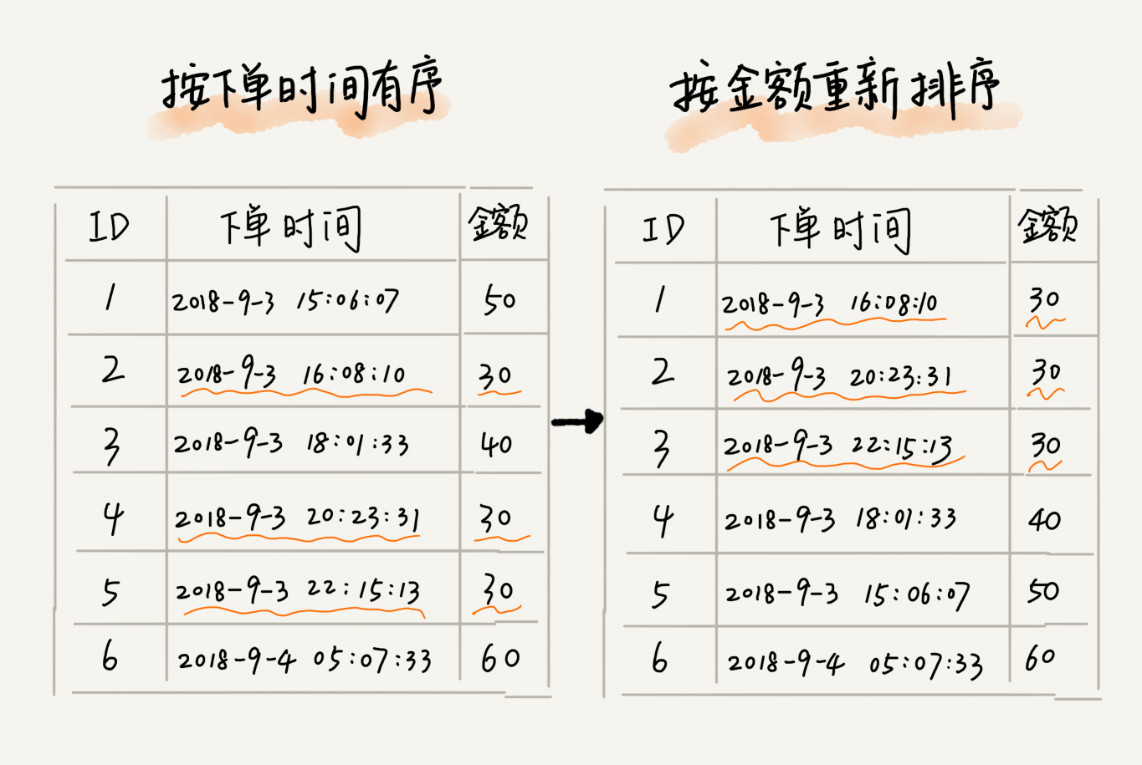## 冒泡排序（Bubble Sort）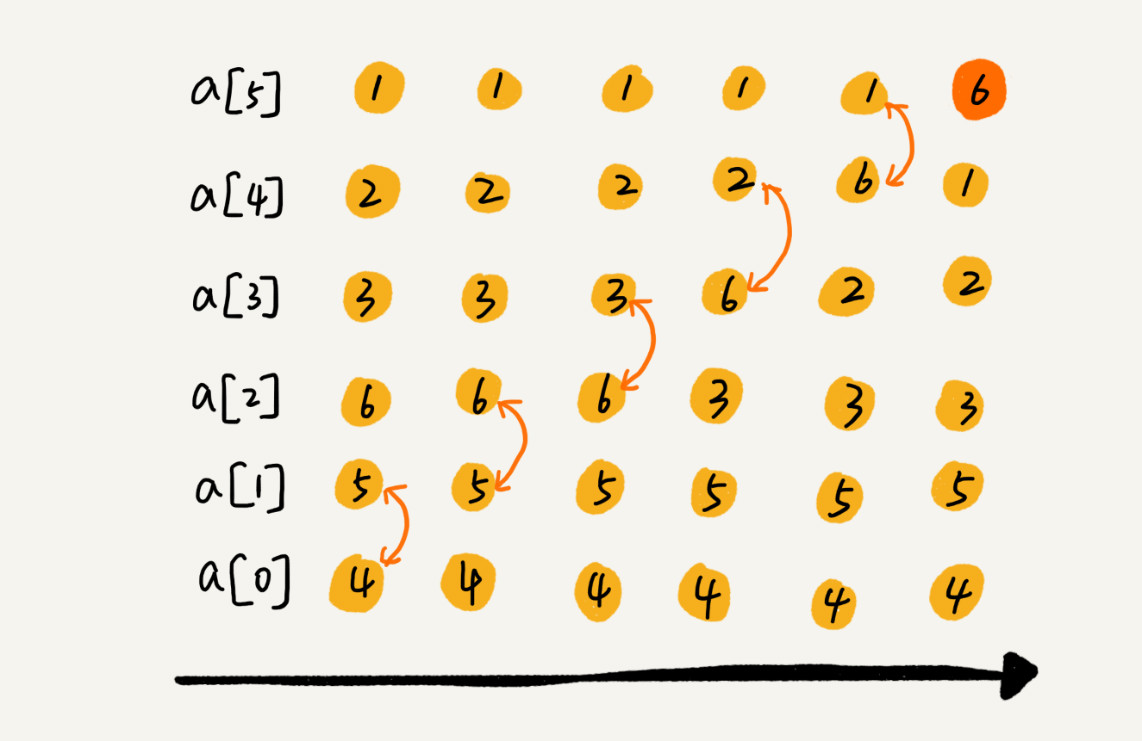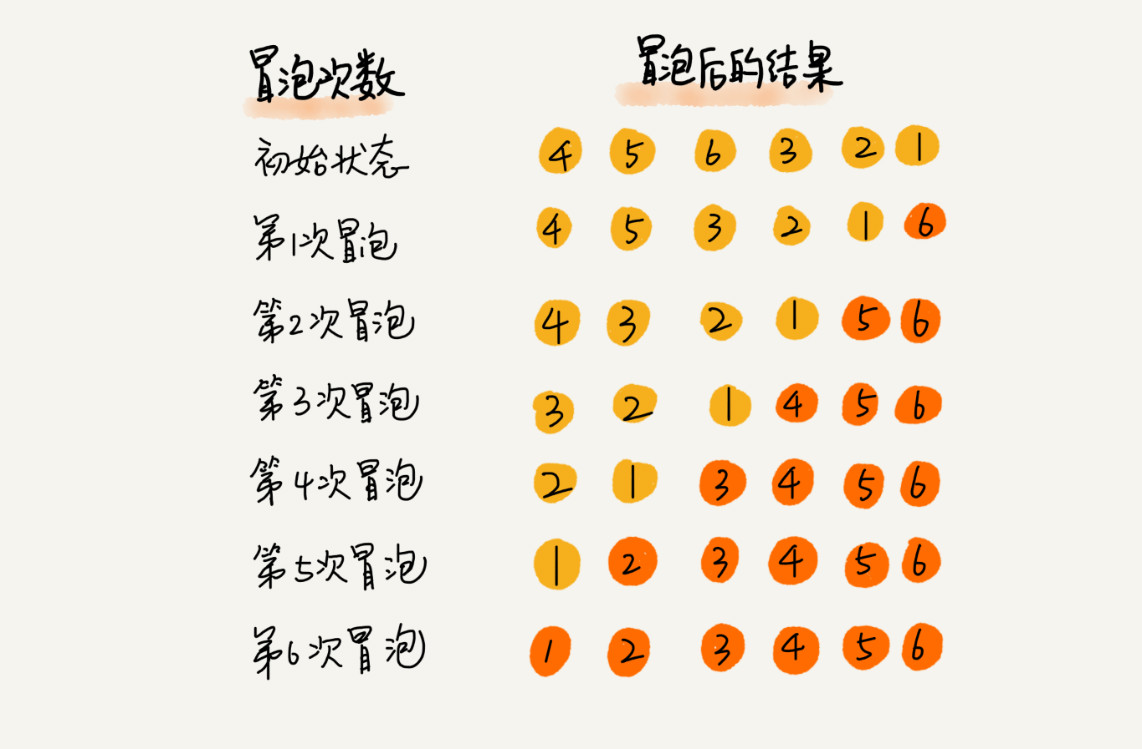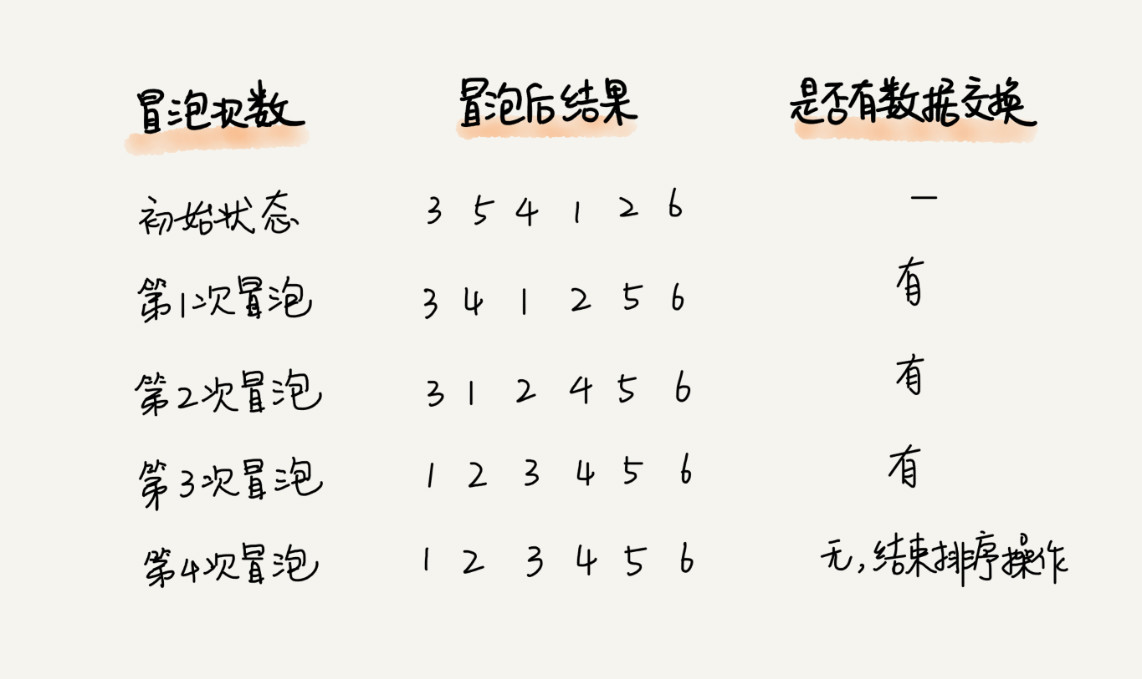``````// 冒泡排序，a 表示数组，n 表示数组大小
public void bubbleSort(int[] a, int n) {
if (n <= 1) return;

for (int i = 0; i < n; ++i) {
// 提前退出冒泡循环的标志位
boolean flag = false;
for (int j = 0; j < n - i - 1; ++j) {
if (a[j] > a[j+1]) { // 交换
int tmp = a[j];
a[j] = a[j+1];
a[j+1] = tmp;
flag = true;  // 表示有数据交换
}
}
if (!flag) break;  // 没有数据交换，提前退出
}
}``````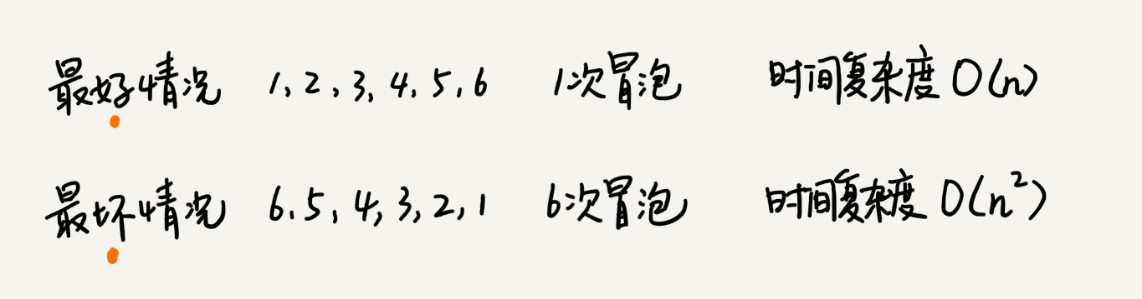``有序元素对：a[i] <= a[j], 如果 i < j。``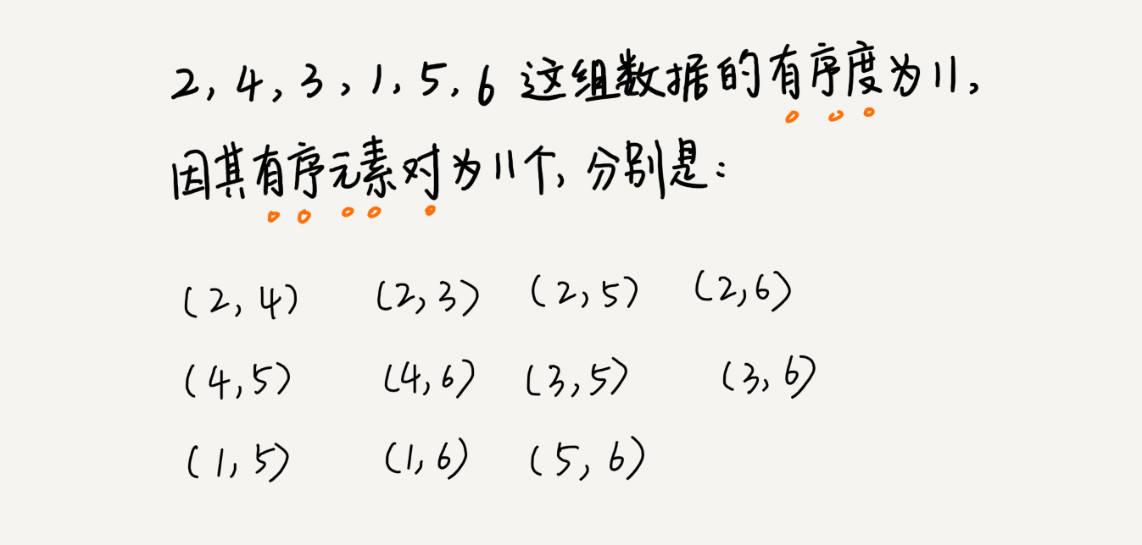``逆序元素对：a[i] > a[j], 如果 i < j。``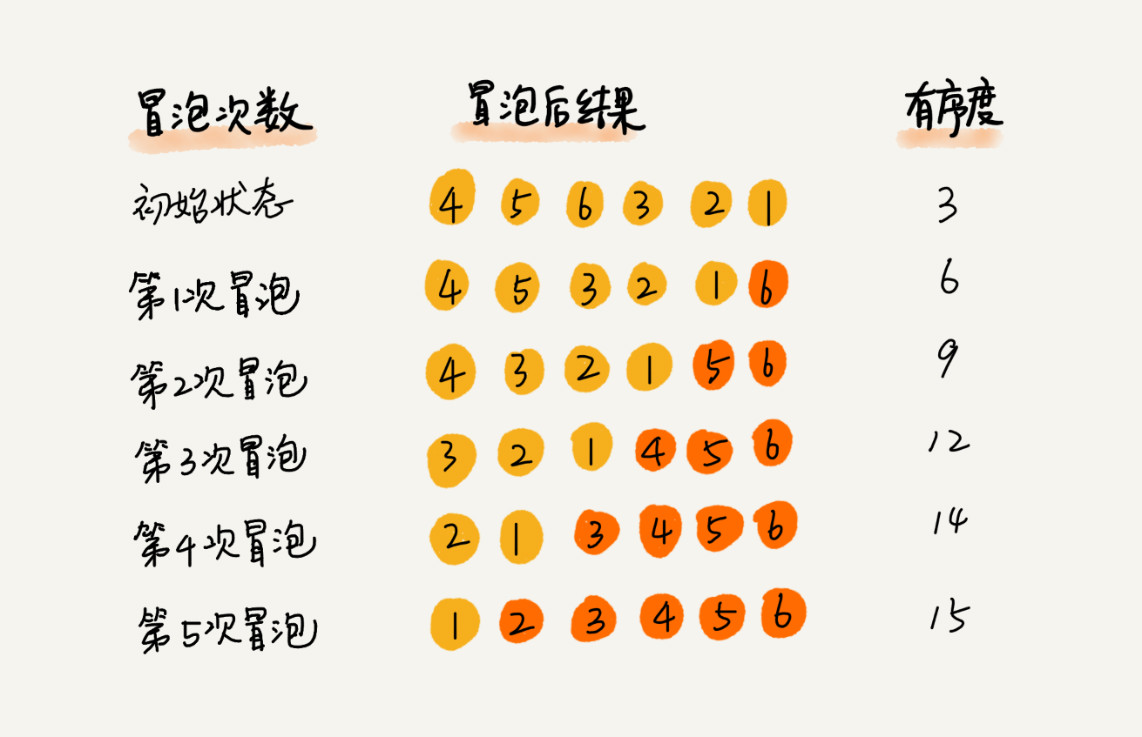## 插入排序（Insertion Sort）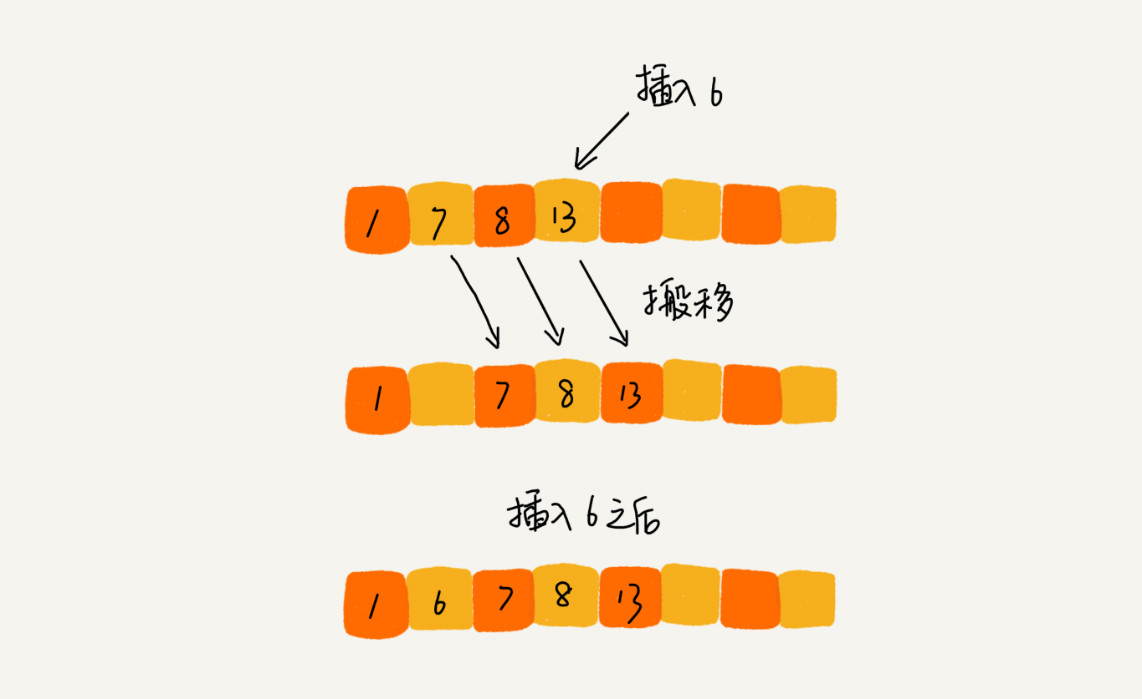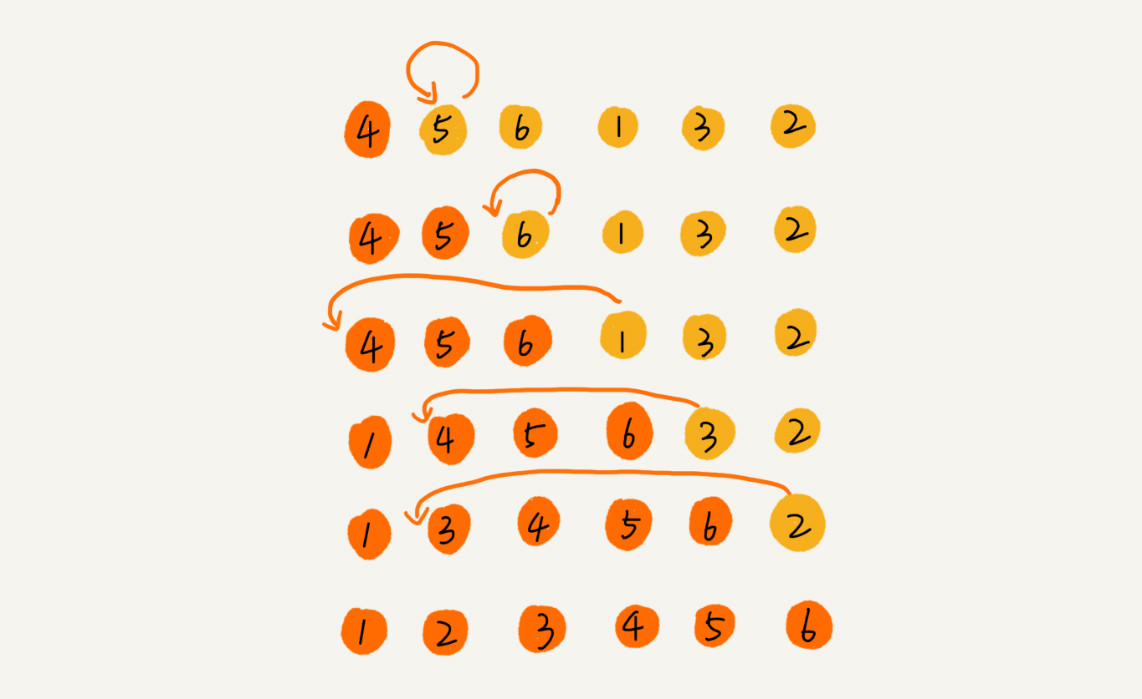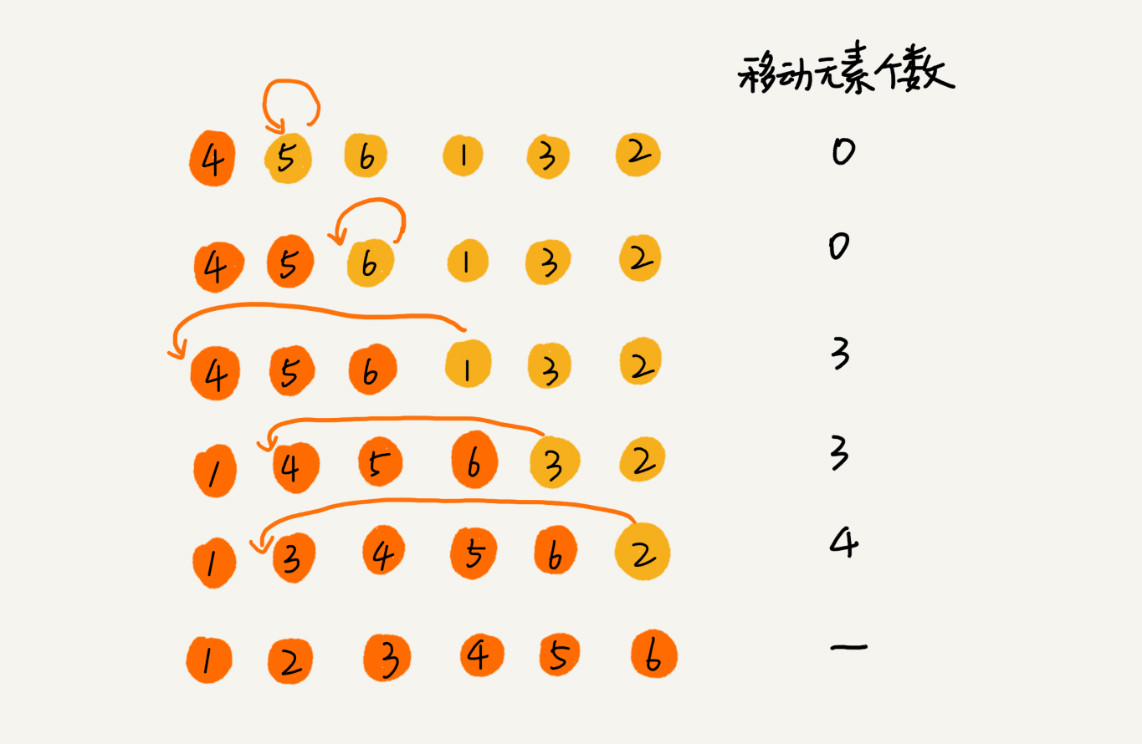``````// 插入排序，a 表示数组，n 表示数组大小
public void insertionSort(int[] a, int n) {
if (n <= 1) return;

for (int i = 1; i < n; ++i) {
int value = a[i];
int j = i - 1;
// 查找插入的位置
for (; j >= 0; --j) {
if (a[j] > value) {
a[j+1] = a[j];  // 数据移动
} else {
break;
}
}
a[j+1] = value; // 插入数据
}
}``````

## 选择排序（Selection Sort）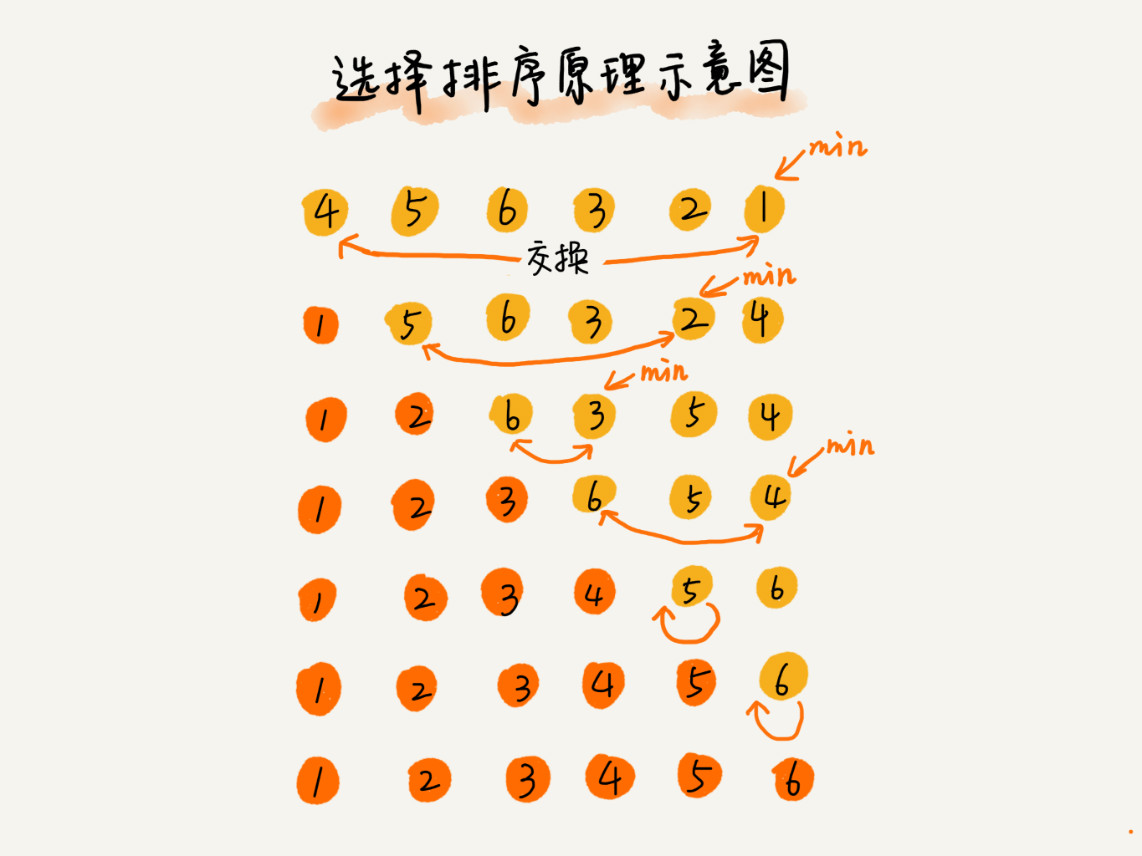## 总结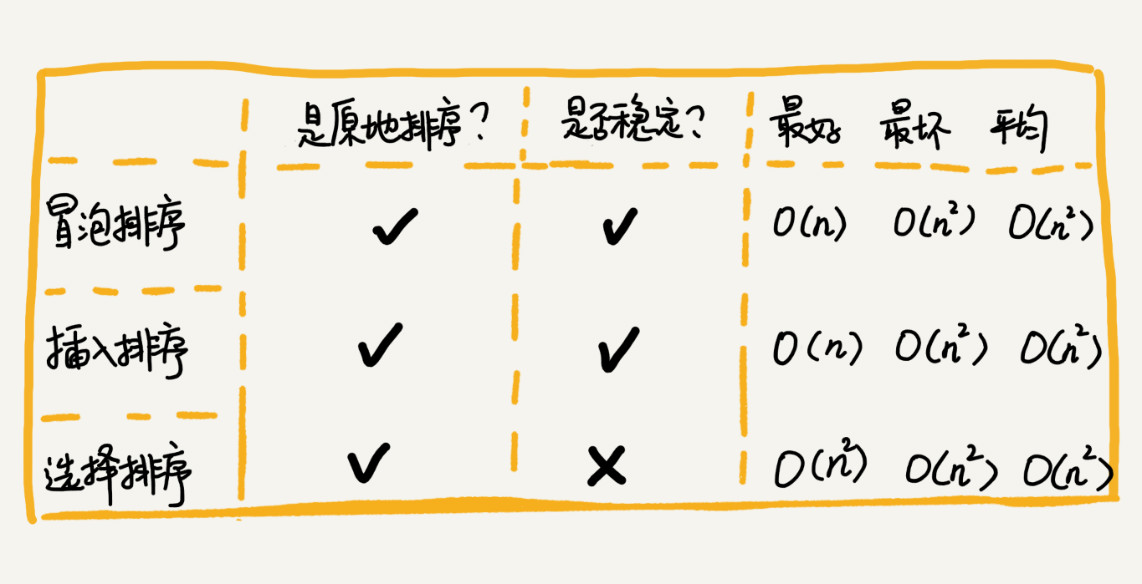## 思考

• 选择排序和插入排序的时间复杂度相同，都是\$O(n^2)\$，在实际的软件开发中，为什么更倾向于使用插入排序而不是冒泡排序算法呢？

前面分析冒泡排序和插入排序的时候讲到，冒泡排序不管怎么优化，元素交换的次数是一个固定值，是原始数据的逆序度。插入排序是同样的，不管怎么优化，元素移动的次数也等于原始数据的逆序度。

但是，从代码实现上来看，冒泡排序的数据交换要比插入排序的数据移动要复杂，冒泡排序需要 `3` 个赋值操作，而插入排序只需要 `1` 个。来看这段操作：

``````冒泡排序中数据的交换操作：
if (a[j] > a[j+1]) { // 交换
int tmp = a[j];
a[j] = a[j+1];
a[j+1] = tmp;
flag = true;
}

插入排序中数据的移动操作：
if (a[j] > value) {
a[j+1] = a[j];  // 数据移动
} else {
break;
}``````

把执行一个赋值语句的时间粗略地计为单位时间（`unit_time`），然后分别用冒泡排序和插入排序对同一个逆序度是 `K` 的数组进行排序。用冒泡排序，需要 `K` 次交换操作，每次需要 `3` 个赋值语句，所以交换操> 作总耗时就是 `3*K` 单位时间。而插入排序中数据移动操作只需要 `K` 个单位时间。

这个只是非常理论的分析，为了实验，针对上面的冒泡排序和插入排序的 `Java` 代码，写了一个性能对比测试程序，随机生成 `10000` 个数组，每个数组中包含 `200` 个数据，然后在机器上分别用冒泡和插入排序算> 法来排序，冒泡排序算法大约 `700ms` 才能执行完成，而插入排序只需要 `100ms` 左右就能搞定！

所以，虽然冒泡排序和插入排序在时间复杂度上是一样的，都是 \$O(n^2)\$，但是如果希望把性能优化做到极致，那肯定首选插入排序。插入排序的算法思路也有很大的优化空间，只是讲了最基础的一种。如果你对插入排> 序的优化感兴趣，可以自行学习一下希尔排序。

• 特定算法是依赖特定的数据结构的。上面的几种排序算法，都是基于数组实现的。如果数据存储在链表中，这三种排序算法还能工作吗？如果能，那相应的时间、空间复杂度又是多少呢？